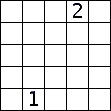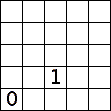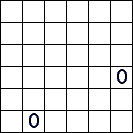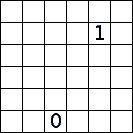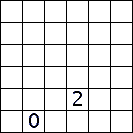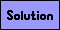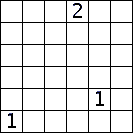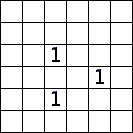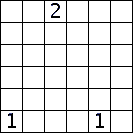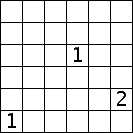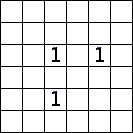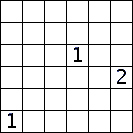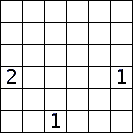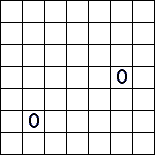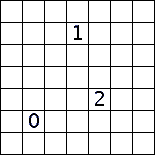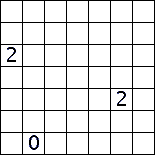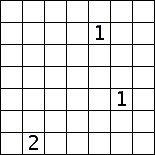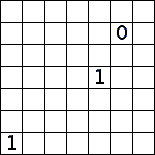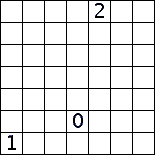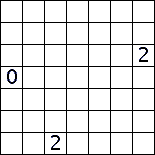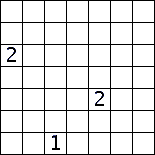Latin Square Puzzles

In each puzzle, shade in some of the squares so that: 1) no shaded squares are adjacent (including diagonally), 2) there is exactly one shaded square in each row and in each column, 3) no square containing a number can be shaded, and 4) each number should be equal to the number of adjacent (including diagonally) shaded squares. Each puzzle has a unique solution.